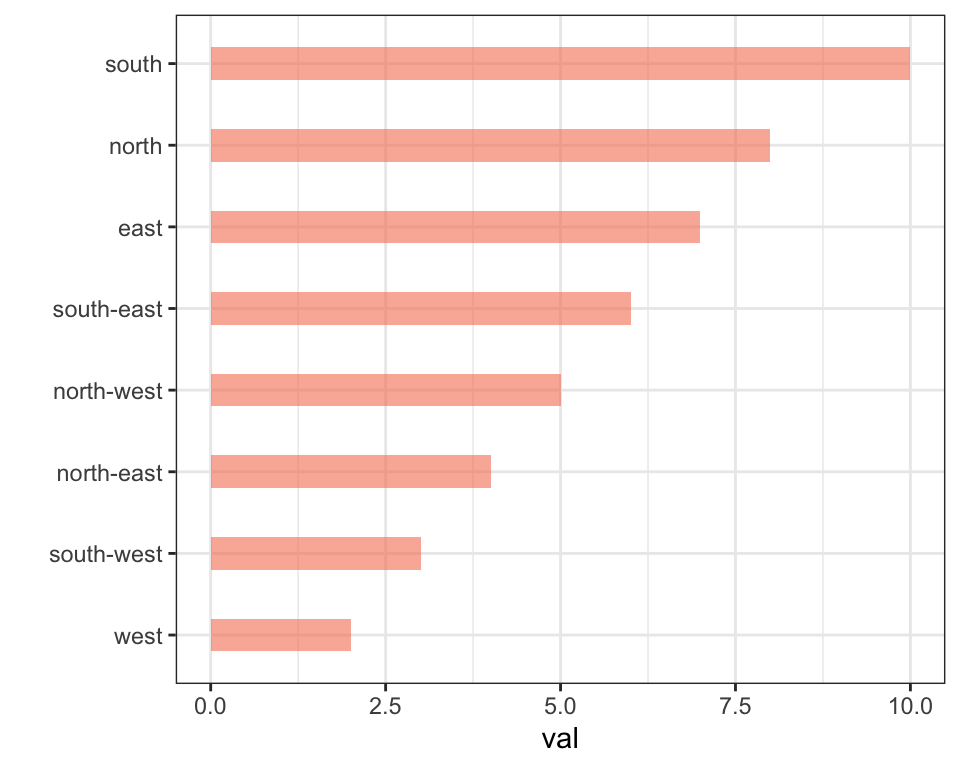# Reorder a variable with ggplot2

This post describes how to reorder a variable in a `ggplot2` chart. Several methods are suggested, always providing examples with reproducible code chunks. Note that this course on data manipulation can be helpful here.

Reordering groups in a `ggplot2` chart can be a struggle. This is due to the fact that ggplot2 takes into account the order of the `factor` levels, not the order you observe in your data frame. You can sort your input data frame with `sort()` or `arrange()`, it will never have any impact on your `ggplot2` output.

This post explains how to reorder the level of your factor through several examples. Examples are based on 2 dummy datasets:

``````# Library
library(ggplot2)
library(dplyr)

# Dataset 1: one value per group
data <- data.frame(
name=c("north","south","south-east","north-west","south-west","north-east","west","east"),
val=sample(seq(1,10), 8 )
)

# Dataset 2: several values per group (natively provided in R)
# mpg``````

# Method 1: the `forcats` library

The forcats library is a library from the tidyverse especially made to handle factors in R. It provides a suite of useful tools that solve common problems with factors. The `fct_reorder()` function allows to reorder the factor (`data\$name` for example) following the value of another column (`data\$val` here).

``````# load the library
library(forcats)

# Reorder following the value of another column:
data %>%
mutate(name = fct_reorder(name, val)) %>%
ggplot( aes(x=name, y=val)) +
geom_bar(stat="identity", fill="#f68060", alpha=.6, width=.4) +
coord_flip() +
xlab("") +
theme_bw()

# Reverse side
data %>%
mutate(name = fct_reorder(name, desc(val))) %>%
ggplot( aes(x=name, y=val)) +
geom_bar(stat="identity", fill="#f68060", alpha=.6, width=.4) +
coord_flip() +
xlab("") +
theme_bw()``````If you have several values per level of your factor, you can specify which function to apply to determine the order. The default is to use the median, but you can use the number of data points per group to make the classification:

``````# Using median
mpg %>%
mutate(class = fct_reorder(class, hwy, .fun='median')) %>%
ggplot( aes(x=reorder(class, hwy), y=hwy, fill=class)) +
geom_boxplot() +
xlab("class") +
theme(legend.position="none") +
xlab("")

# Using number of observation per group
mpg %>%
mutate(class = fct_reorder(class, hwy, .fun='length' )) %>%
ggplot( aes(x=class, y=hwy, fill=class)) +
geom_boxplot() +
xlab("class") +
theme(legend.position="none") +
xlab("") +
xlab("")``````The last common operation is to provide a specific order to your levels, you can do so using the `fct_relevel()` function as follow:

``````# Reorder following a precise order
p <- data %>%
mutate(name = fct_relevel(name,
"north", "north-east", "east",
"south-east", "south", "south-west",
"west", "north-west")) %>%
ggplot( aes(x=name, y=val)) +
geom_bar(stat="identity") +
xlab("")
#p``````# Method 2: using `dplyr` only

The `mutate()` function of `dplyr` allows to create a new variable or modify an existing one. It is possible to use it to recreate a factor with a specific order. Here are 2 examples:

• The first use `arrange()` to sort your data frame, and reorder the factor following this desired order.
• The second specifies a custom order for the factor giving the levels one by one.
``````data %>%
arrange(val) %>%    # First sort by val. This sort the dataframe but NOT the factor levels
mutate(name=factor(name, levels=name)) %>%   # This trick update the factor levels
ggplot( aes(x=name, y=val)) +
geom_segment( aes(xend=name, yend=0)) +
geom_point( size=4, color="orange") +
coord_flip() +
theme_bw() +
xlab("")

data %>%
arrange(val) %>%
mutate(name = factor(name, levels=c("north", "north-east", "east", "south-east", "south", "south-west", "west", "north-west"))) %>%
ggplot( aes(x=name, y=val)) +
geom_segment( aes(xend=name, yend=0)) +
geom_point( size=4, color="orange") +
theme_bw() +
xlab("")``````# Method 3: the `reorder()` function of base R

In case your an unconditional user of the good old R, here is how to control the order using the `reorder()` function inside a `with()` call:

``````# reorder is close to order, but is made to change the order of the factor levels.
mpg\$class = with(mpg, reorder(class, hwy, median))

p <- mpg %>%
ggplot( aes(x=class, y=hwy, fill=class)) +
geom_violin() +
xlab("class") +
theme(legend.position="none") +
xlab("")
#p``````Related chart types

## Contact

This document is a work by Yan Holtz. Any feedback is highly encouraged. You can fill an issue on Github, drop me a message on Twitter, or send an email pasting yan.holtz.data with gmail.com.